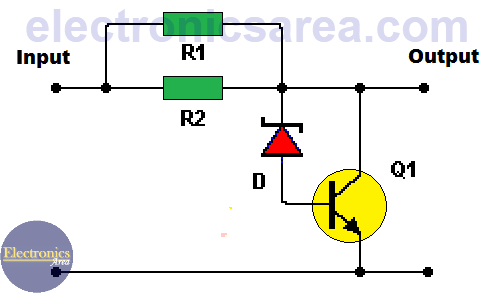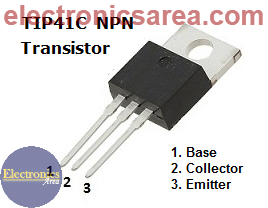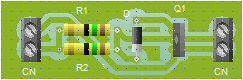# 12V to 9V DC Converter

This 12V to 9V DC converter is very useful to power 9V DC devices in a car that uses a 12V DC battery. The maximum current consumption allowed by this circuit is 0.8 amps. This 12V to 9V DC converter is implemented using a zener diode and a NPN bipolar transistor (Q1) as shown in the circuit diagram.

To better understand the operation of this circuit, the following article is recommended: Zener voltage regulator.

## How the 12V to 9V DC Converter works?

As in the zener voltage regulator from the above suggested link, a series resistor is used (R1 and R2 are used in parallel on this case).

The output voltage of the circuit is the zener diode voltage plus the transistor base – emitter voltage. (Vout = Vz + Vbe). Vout = 8.2 + 0.7 = 8.9 volts.  A greater variation of the output current is allowed using the NPN transistor. This is not the case when the zener diode is used alone.Notes:

• Use a heatsink as large as possible for thw Q1 transistor.
• The converter input voltage must not exceed 14 Volts.TIP41C NPN Bipolar Transistor

## List of components for the 12V to 9V DC converter

• 1  TIP41C NPN bipolar transistor or similar (Q1)
• 2  6.8 ohm resistors (5 watts or more) (R1, R2)
• 1  1N756 zener diode 8.2 volts or similar (D)
• 1 heatsink to be used on the Q1 transistor (required)

## Suggested 12V to 9V DC converter printed circuit board

The following diagrams shows a suggested printed circuit board (PCB) and an approximate view of the final circuit appearance.• 4
•
•
•
•
•
•
•
•
4
Shares
•
4
Shares
• 4
•
•
•
•
•
•
•
Tagged with: ,